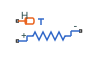# Thermal Resistor

Resistor with thermal port

•Libraries:
Simscape / Foundation Library / Electrical / Electrical Elements

## Description

The Thermal Resistor block represents a temperature-dependent resistor. When the temperature at the thermal port is T, the resistance is

`$R={R}_{0}\left(1+\alpha \left(T-{T}_{0}\right)\right)$`

where:

• R0 is the nominal resistance at the reference temperature T0.

• α is the temperature coefficient.

The following equation describes the thermal behavior of the block:

`$Q={K}_{d}{t}_{c}\frac{dT}{dt}-{i}^{2}R$`

where:

• Q is the net heat flow into port H.

• Kd is the Dissipation factor parameter value.

• tc is the Thermal time constant parameter value.

• dT/dt is the rate of change of the temperature.

• i is the current through the resistor.

### Variables

To set the priority and initial target values for the block variables prior to simulation, use the Initial Targets section in the block dialog box or Property Inspector. For more information, see Set Priority and Initial Target for Block Variables.

Nominal values provide a way to specify the expected magnitude of a variable in a model. Using system scaling based on nominal values increases the simulation robustness. Nominal values can come from different sources, one of which is the Nominal Values section in the block dialog box or Property Inspector. For more information, see Modify Nominal Values for a Block Variable.

In particular, the Temperature variable lets you set a high-priority target for the temperature of the thermal resistor at the start of the simulation. The default value is `300` K.

## Ports

### Conserving

expand all

Thermal conserving port that provides the resistor temperature.

Electrical conserving port associated with the resistor positive terminal.

Electrical conserving port associated with the resistor negative terminal.

## Parameters

expand all

The nominal resistance of the thermistor at the reference temperature. Many datasheets quote the nominal resistance at 25°C (298.15 K) and list it as R25.

The temperature at which the nominal resistance was measured.

The coefficient α in the equation that describes resistance as a function of temperature.

The time it takes the resistor temperature to reach 63% of the final temperature change when a step change in ambient temperature occurs.

The thermal power required to raise the thermal resistor temperature by one K.

## Version History

Introduced in R2016a